/  Technology   /  All about Categorical Variable Encoding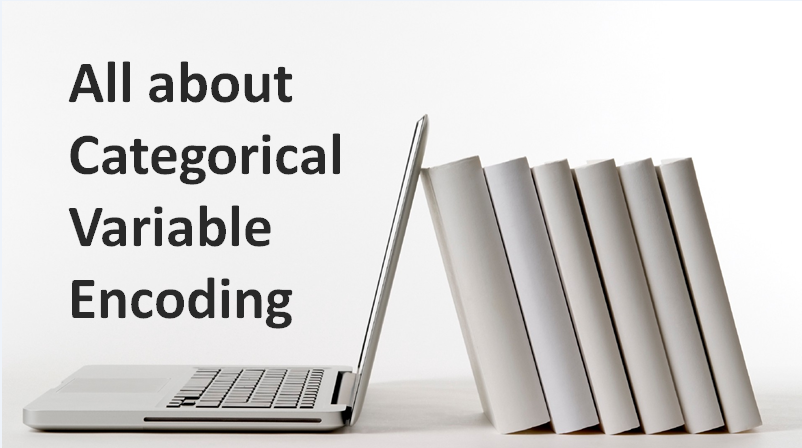## All about Categorical Variable Encoding

The performance of a machine learning model not only depends on the model and the hyperparameters but also on how we process and feed different types of variables to the model. Most of the Machine learning algorithms cannot handle categorical variables unless we convert them to numerical values.

A typical data scientist spends 70 – 80% of his time cleaning and preparing the data. And converting categorical data is an unavoidable activity. It not only elevates the model quality but also helps in better feature engineering.

In this article, I will be discussing various types of categorical data encoding methods with implementation in Python.

## What is categorical data?

Categorical variables can be divided into two categories:

• Ordinal Data:The categories have particular order
• Nominal Data:The categories do not have any order

Example of Ordinal variables:

• High, Medium, Low
• “Strongly agree,” Agree, Neutral, Disagree, and “Strongly Disagree.”

In Ordinal data, while encoding, one should ensure the encoding of variables retains the information regarding the order in which the category is provided.

Few examples as below for Nominal variable:

• Red, Yellow, Pink, Blue
• Singapore, Japan, USA, India, Korea
• Cow, Dog, Cat, Snake

While encoding Nominal data, we have to think about the presence or absence of a feature.

There are several ways we can encode these categorical variables as numbers and use them in an algorithm.

For encoding categorical data, we have a python package called category_encoders. The subsequent code helps how to install.

`pip install category_encoders`

## Label Encoding or Ordinal Encoding

In this encoding technique, each category is allocated a value from 1 through N (here N is the number of categories for the feature). One major issue with this approach is that the algorithm might consider them as some order, or there is some relationship between classes even though there is no relation or order between these classes.

```import category_encoders as ce
import pandas as pd
train_df=pd.DataFrame({'Degree':['High school','Masters','Diploma','Bachelors','Bachelors','Masters','Phd','High school','High school']})
#create object of Ordinalencoding
encoder= ce.OrdinalEncoder(cols=['Degree'],return_df=True,
mapping=[{'col':'Degree',
'mapping':{'None':0,'High school':1,'Diploma':2,'Bachelors':3,'Masters':4,'phd':5}}])
#Original data
train_df``````#fit and transform train data
df_train_transformed = encoder.fit_transform(train_df)```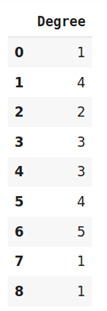## One Hot Encoding

When the features are nominal(do not have any order) we use this categorical data encoding technique. In one hot encoding, we create a new variable for each level of a categorical feature. Each category is mapped with a binary variable   comprising either 0 or 1. Here, 0 represents the absence, and 1 represents the presence of that category.

These newly created binary features are known as Dummy variables which depends on the levels existing in the categorical variable. Let us take an example to know this better. Suppose we have a dataset with a category animal, having different animals like Dog, Cat, Sheep, Cow, Lion. Now we have to one-hot encode this data.The second table represents dummy variables after encoding each representing a category in the feature Animal. Now for each category that is present, we have 1 in the column of that category and 0 for the others. Let’s see how to work on one-hot encoding in python.

```import category_encoders as ce
import pandas as pd
]})
#Create object for one-hot encoding
encoder=ce.OneHotEncoder(cols='City',handle_unknown='return_nan',return_df=True,use_cat_names=True)
#Original Data
data```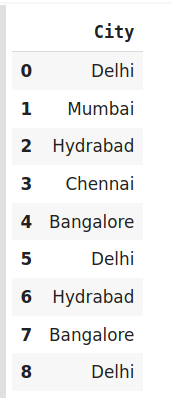```#Fit and transform Data
data_encoded = encoder.fit_transform(data)
data_encoded```## Dummy Encoding

Dummy coding scheme is like one-hot encoding. This categorical data encoding method converts the categorical variable into a group of binary variables (also referred to as dummy variables). In the case of one-hot encoding, it uses N binary variables, for N categories in a variable. The dummy encoding may be a small enhancement over one-hot-encoding. Dummy encoding uses N-1 features to signify N labels/categories.

To understand this better let’s see the image below. Here we are coding an equivalent data using both one-hot encoding and dummy encoding techniques. While one-hot uses 3 variables to represent the data whereas dummy encoding uses 2 variables to code 3 categories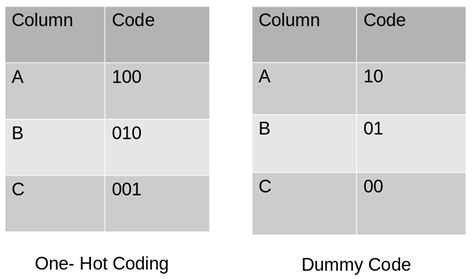Let us implement it in python.

```import category_encoders as ce
import pandas as pd
#Original Data
data```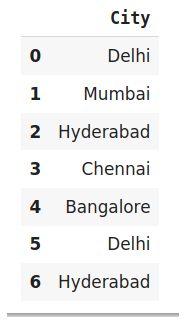```#encode the data
data_encoded=pd.get_dummies(data=data,drop_first=True)
data_encoded```## Effect Encoding

This encoding technique is also referred to as Deviation Encoding or Sum Encoding. Effect encoding is nearly similar to dummy encoding, with a slight difference. In effect encoding, we use three values i.e. 1,0, and -1 whereas in dummy coding, we use 0 and 1 to represent the data.

The row comprising of only 0s in dummy encoding is encoded as -1 in effect encoding.  In the dummy encoding example, the city Bangalore at index 4 was encoded as 0000, but whereas in effect encoding it is represented by -1-1-1-1.

Let us see how we implement it in python-

```import category_encoders as ce
import pandas as pd
encoder=ce.sum_coding.SumEncoder(cols='City',verbose=False,)
#Original Data
data````encoder.fit_transform(data)`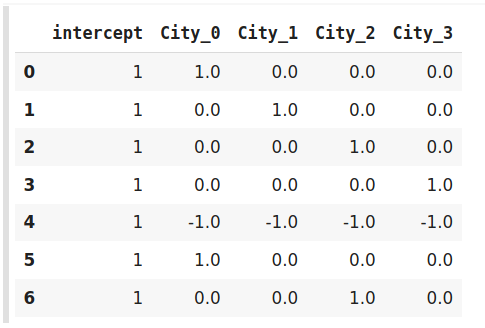## Hash Encoder

To understand Hash encoding it is necessary to understand about hashing. Hashing is the transformation of arbitrary size input in the form of a fixed-size value. We use hashing algorithms to perform hashing operations i.e to generate the hash value of an input. Further, hashing is a one-way process, in other words, one cannot generate original input from the hash representation.

Hashing has numerous applications like data retrieval, checking data corruption, and in data encryption also. We have multiple hash functions available for example Message Digest (MD, MD2, MD5), Secure Hash Function (SHA0, SHA1, SHA2), and many more.

The Hash encoder represents categorical features using the new dimensions which is same as one-hot encoding. Here, the user can assign the number of dimensions after transformation using n_component argument. For example – A feature with 5 categories can be represented using N new features likewise, a feature with 100 categories can also be transformed using N new features.

By default, the Hashing encoder uses the md5 hashing algorithm but a user can pass any algorithm of his choice

```import category_encoders as ce
import pandas as pd
#Create the dataframe
data=pd.DataFrame({'Month':['January','April','March','April','Februay','June','July','June','September']})
#Create object for hash encoder
encoder=ce.HashingEncoder(cols='Month',n_components=6)``````#Fit and Transform Data
encoder.fit_transform(data)

```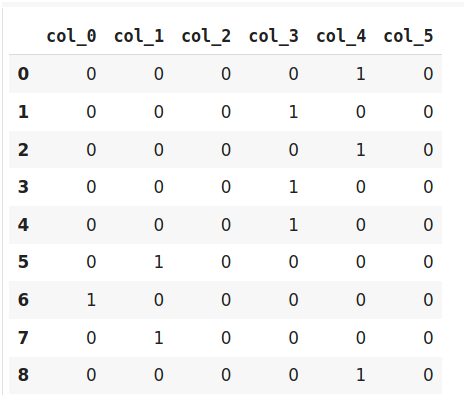Since Hashing transforms the data in lesser dimensions, it may lead to loss of information. Another issue faced by hashing encoder is that the collision. Since here, a large number of features are depicted into lesser dimensions, hence multiple values can be represented by the same hash value, this is known as a collision.

Moreover, hashing encoders are very successful in some Kaggle competitions. It is great to attempt if the dataset has high cardinality features.

## Binary Encoding

Binary encoding converts a category into binary digits. Each binary digit creates one feature column. If there are n unique categories, then binary encoding marks in the only log(base 2)ⁿ features. If we have four features; then the total number of the binary encoded features will be three features. Compared to One Hot Encoding, this will require less feature columns (for 100 categories One Hot Encoding will have 100 features while for Binary encoding, we will need just seven features).

```#Import the libraries
import category_encoders as ce
import pandas as pd
#Create the Dataframe
#Create object for binary encoding
encoder= ce.BinaryEncoder(cols=['city'],return_df=True)
#Original Data
data```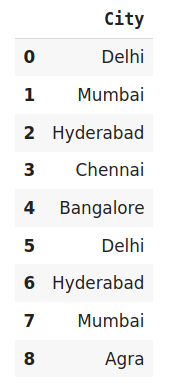```#Fit and Transform Data
data_encoded=encoder.fit_transform(data)
data_encoded```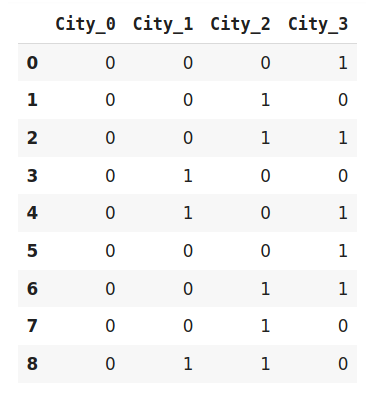## Target Encoding

Target encoding is a Baysian encoding technique.

In target encoding, we compute the mean of the target variable for each category and replace the category variable with the mean value. In the case of the categorical target variables, the posterior probability of the target replaces each category.

```#import the libraries
import pandas as pd
import category_encoders as ce
#Create the Dataframe
data=pd.DataFrame({'class':['A,','B','C','B','C','A','A','A'],'Marks':[50,30,70,80,45,97,80,68]})
#Create target encoding object
encoder=ce.TargetEncoder(cols='class')
#Original Data
Data```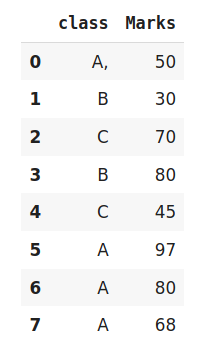```#Fit and Transform Train Data
encoder.fit_transform(data['class'],data['Marks'])```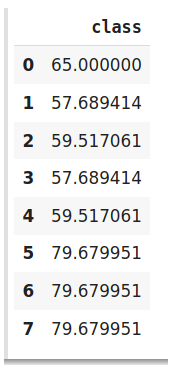We perform Target encoding for train data only and code the test data using results obtained from the training dataset. Although, a very efficient coding system, it has few issues responsible for deteriorating the model performance.

To summarize, encoding categorical data is an unavoidable part of the feature engineering. It is more important to know what coding scheme should we use. Having into consideration the dataset we are working with and the model we are going to use.